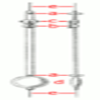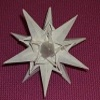# Resources tagged with: Differential equations

Filter by: Content type:
Age range:
Challenge level:

### There are 13 results

Broad Topics > Calculus > Differential equations### The Not-so-simple Pendulum 2

##### Age 16 to 18 Challenge Level:

Things are roughened up and friction is now added to the approximate simple pendulum### Differential Equation Matcher

##### Age 16 to 18 Challenge Level:

Match the descriptions of physical processes to these differential equations.### The Real Hydrogen Atom

##### Age 16 to 18 Challenge Level:

Dip your toe into the world of quantum mechanics by looking at the Schrodinger equation for hydrogen atoms### It's Only a Minus Sign

##### Age 16 to 18 Challenge Level:

Solve these differential equations to see how a minus sign can change the answer### Bessel's Equation

##### Age 16 to 18 Challenge Level:

Get further into power series using the fascinating Bessel's equation.### The Not-so-simple Pendulum 1

##### Age 16 to 18 Challenge Level:

See how the motion of the simple pendulum is not-so-simple after all.### Taking Trigonometry Series-ly

##### Age 16 to 18 Challenge Level:

Look at the advanced way of viewing sin and cos through their power series.### Earth Orbit

##### Age 16 to 18 Challenge Level:

Follow in the steps of Newton and find the path that the earth follows around the sun.### Modelling Assumptions in Mechanics

##### Age 16 to 18

An article demonstrating mathematically how various physical modelling assumptions affect the solution to the seemingly simple problem of the projectile.### Predator - Prey Systems

##### Age 16 to 18 Challenge Level:

See how differential equations might be used to make a realistic model of a system containing predators and their prey.### Reaction Rates

##### Age 16 to 18 Challenge Level:

Explore the possibilities for reaction rates versus concentrations with this non-linear differential equation### What's My Equation?

##### Age 16 to 18 Challenge Level:

Can you find the differential equations giving rise to these famous solutions?### Bird-brained

##### Age 16 to 18 Challenge Level:

How many eggs should a bird lay to maximise the number of chicks that will hatch? An introduction to optimisation.